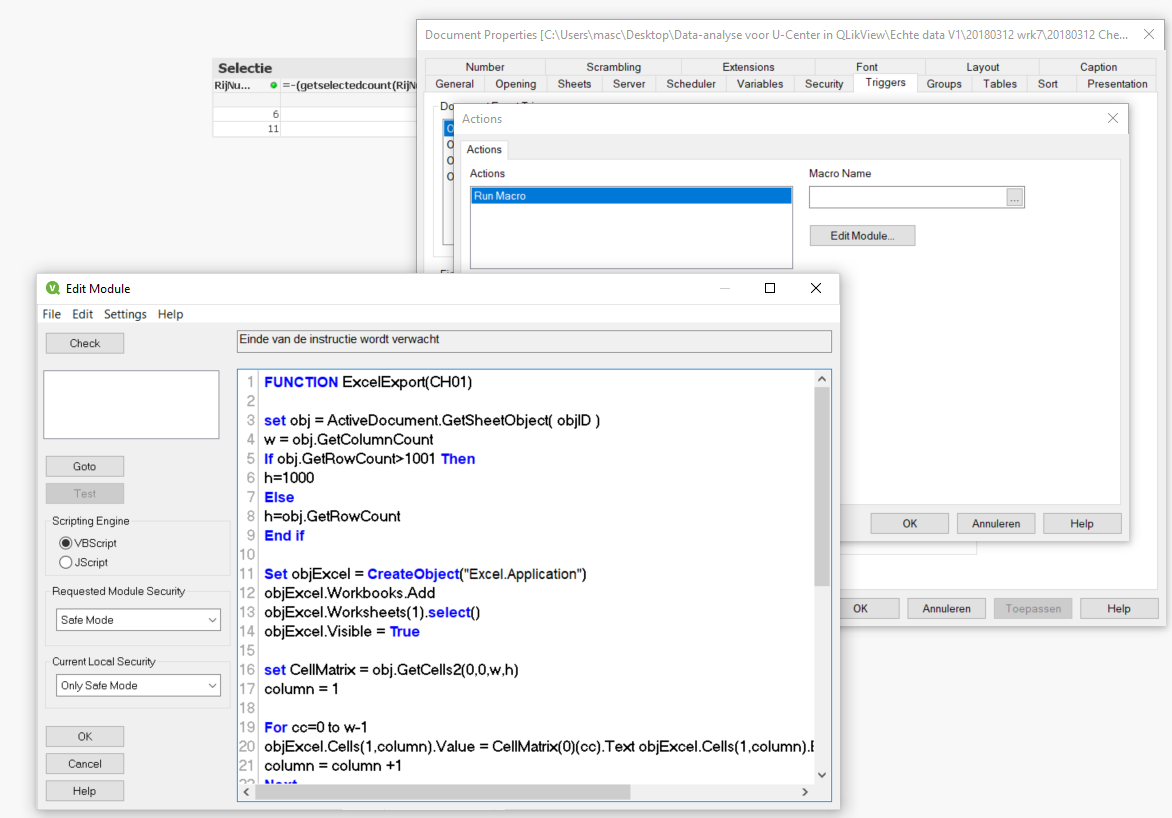# QlikView App Development

Discussion Board for collaboration related to QlikView App Development.

Highlighted
New Contributor III

## Run VBscript as Macro to safe the result of a selection automatically to a file

I tried to safe the result of a selection in a list box automatically to an external file (preferred QVD file and if not possible XLS file). I followed the topics:

https://community.qlik.com/docs/DOC-4870

My list boxMy straight tableThe content of the straight table needs to be stored in an external files automatically after pressing a button. The first problem that I have is that the example code of the topic described before doesn't work.Unfortunately, I never programmed in VBscript so does somebody know why the code doesn't work.

The code in text format is :

FUNCTION ExcelExport(CH01)

set obj = ActiveDocument.GetSheetObject( objID )

w = obj.GetColumnCount

If obj.GetRowCount>1001 Then

h=1000

Else

h=obj.GetRowCount

End if

Set objExcel = CreateObject("Excel.Application")

objExcel.Worksheets(1).select()

objExcel.Visible = True

set CellMatrix = obj.GetCells2(0,0,w,h)

column = 1

For cc=0 to w-1

objExcel.Cells(1,column).Value = CellMatrix(0)(cc).Text objExcel.Cells(1,column).EntireRow.Font.Bold = True

column = column +1

Next

c = 1

r =2

For RowIter=1 to h-1

For ColIter=0 to w-1

objExcel.Cells(r,c).Value = CellMatrix(RowIter)(ColIter).Text

c = c +1

Next

r = r+1 c = 1

Next

END FUNCTION

SUB CallExample ExcelExport("CH01")

END SUB

Tags (2)